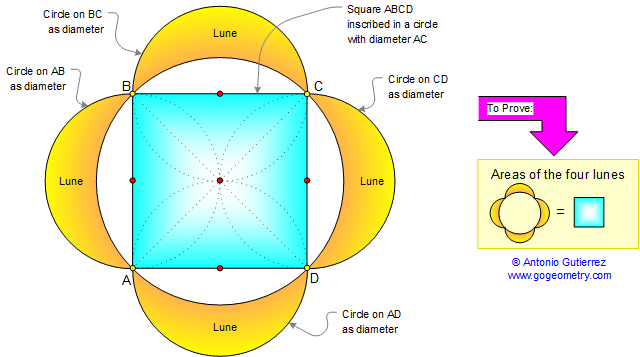# Lune of Hippocrates 5: Squaring the Circle?

In the figure below, the sum of the areas of the four lunes equals the area of the square.

A lune is a plane figure bounded by two circular arcs of unequal radii.

The fact that the areas bounded by arcs were independent of Pi (π) led Hippocrates to continue the hopeless search for a method of squaring the circle.Hippocrates of Chios (c.470 BC - c.410 BC) was a Greek mathematician who worked on the classical problems of squaring the circle and duplicating the cube.

Squaring the circle or the quadrature of the circle  is one of the three great problems of Classical Geometry. It is the challenge to construct a square with the same area as a given circle by using only a finite number of steps with compass and straightedge.

See also: Hippocrates 1, Hippocrates 2, Hippocrates 3, Hippocrates 4, Pythagoras and Circle Area, Similar shapes.

Home | Geometry | Pythagoras Index | Right Triangle Formulas | Lune Index | Square area | Email | By Antonio Gutierrez